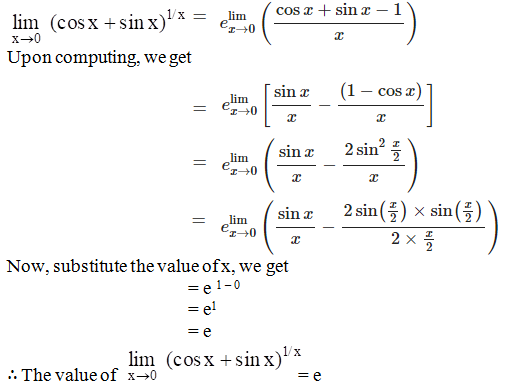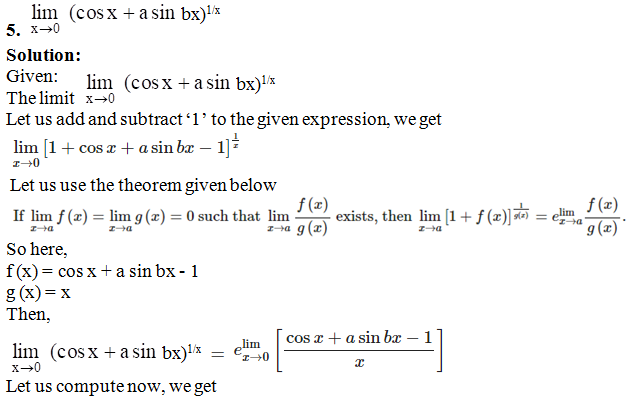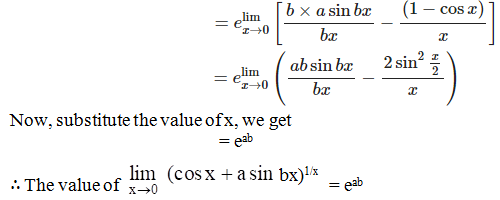# RD Sharma Solutions for Class 11 Chapter 29 - Limits Exercise 29.11

Here, in this exercise, we shall study the concept of evaluation of limits of the form $$1^{\infty }$$ with suitable examples to understand the concepts. Students facing any difficulties in understanding concepts of this exercise can refer to RD Sharma Class 11 Maths Solutions, which is developed by subject matter experts at BYJU’S. All the solutions are created with an aim to boost confidence among students for their exam preparations. The resource also improves problem-solving skills of the students, which are essential from the exam point of view. The PDF of RD Sharma Solutions for Class 11 Maths Chapter 29 Exercise 29.11 can be accessed, from the links given below.

## Download the pdf of RD Sharma Solutions for Class 11 Maths Exercise 29.11 Chapter 29 – Limits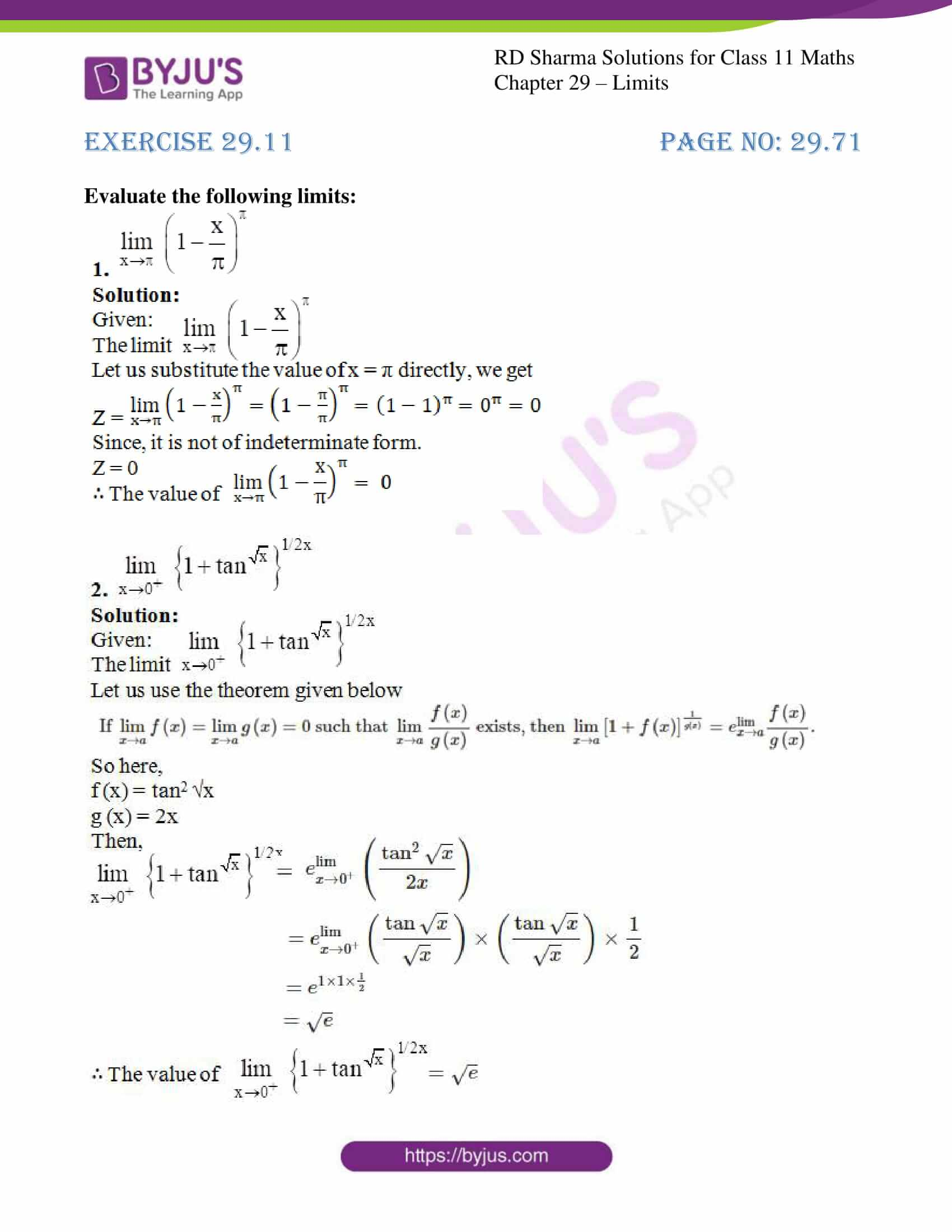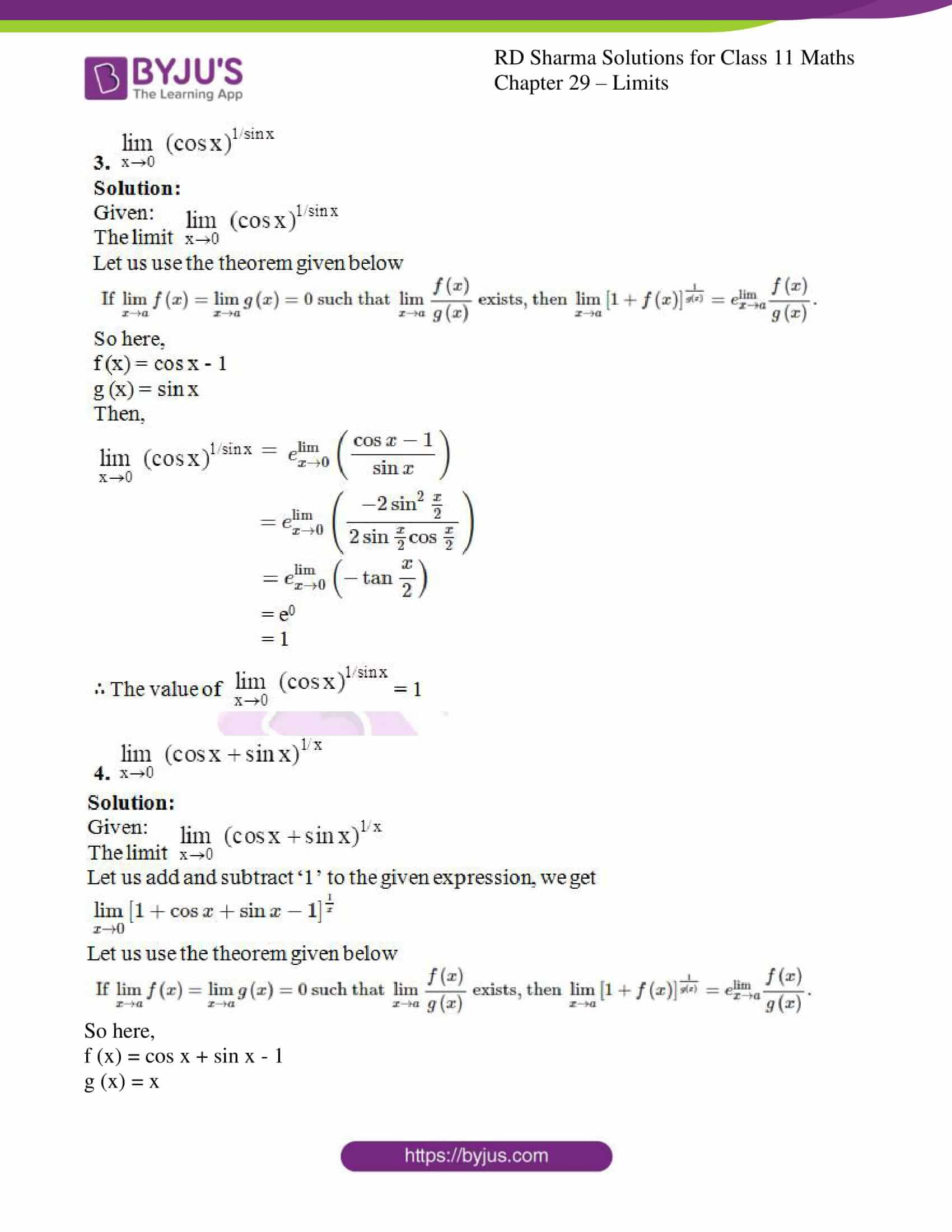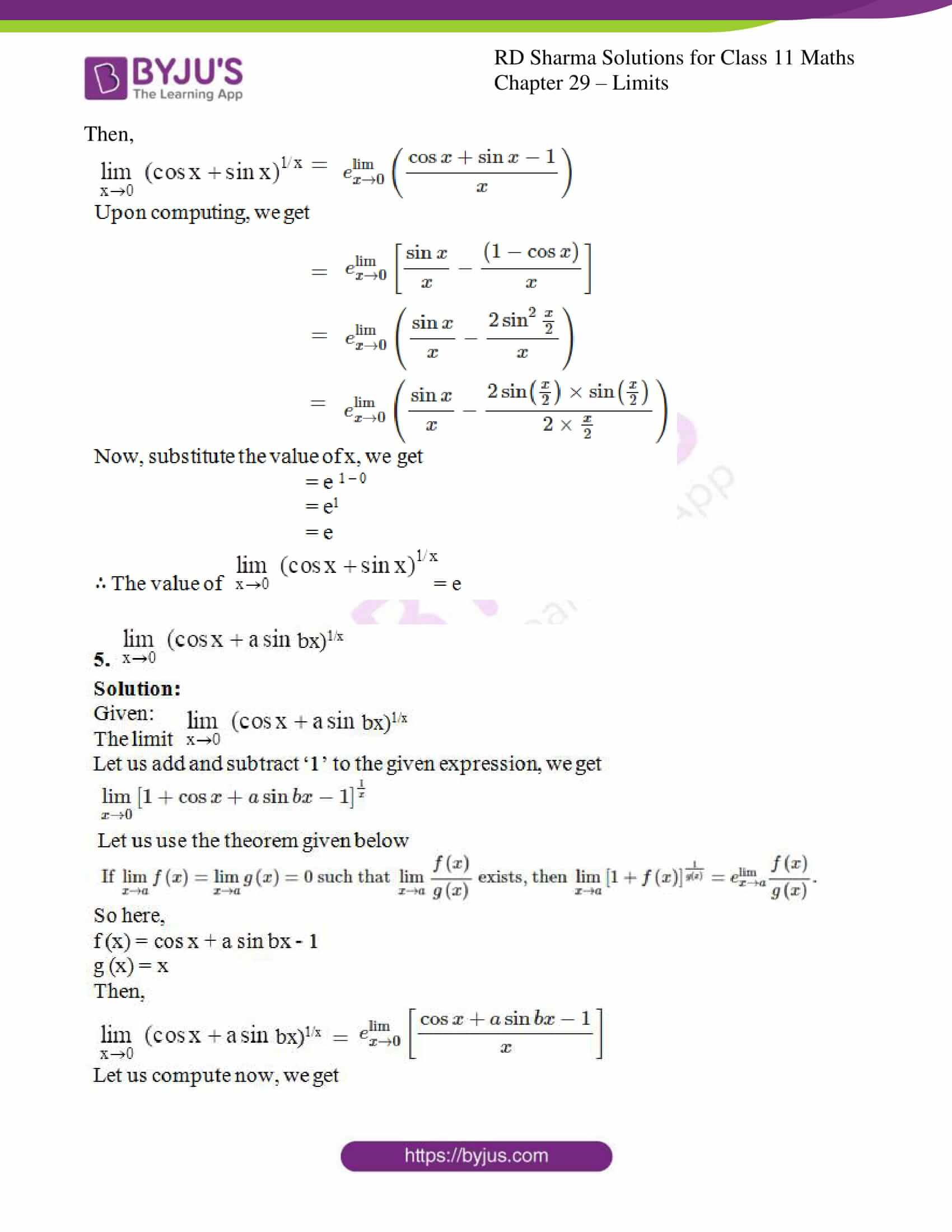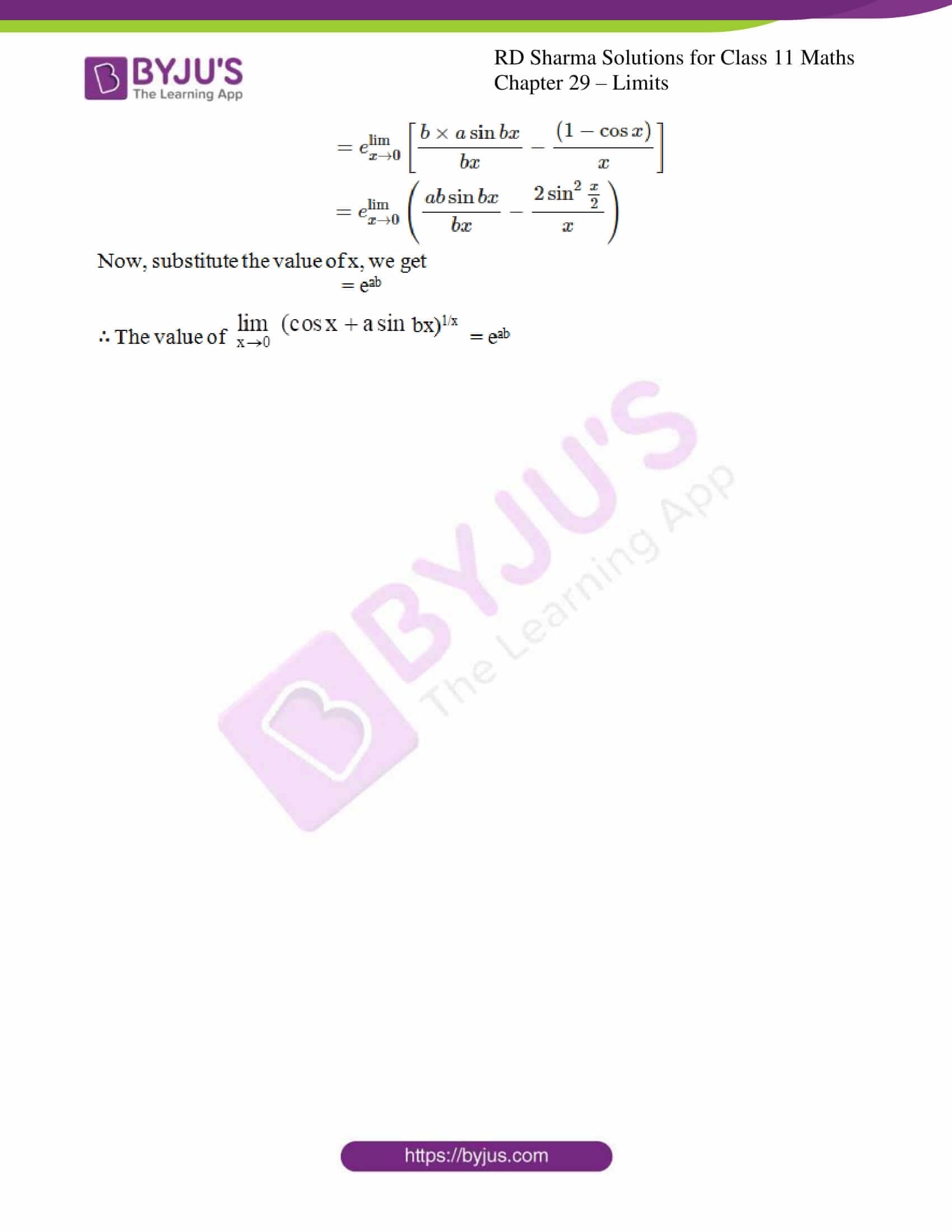### Also, access other exercises of RD Sharma Solutions for Class 11 Maths Chapter 29 – Limits

Exercise 29.1 Solutions

Exercise 29.2 Solutions

Exercise 29.3 Solutions

Exercise 29.4 Solutions

Exercise 29.5 Solutions

Exercise 29.6 Solutions

Exercise 29.7 Solutions

Exercise 29.8 Solutions

Exercise 29.9 Solutions

Exercise 29.10 Solutions

### Access answers to RD Sharma Solutions for Class 11 Maths Exercise 29.11 Chapter 29 – Limits

#### EXERCISE 29.11 PAGE NO: 29.71

Evaluate the following limits: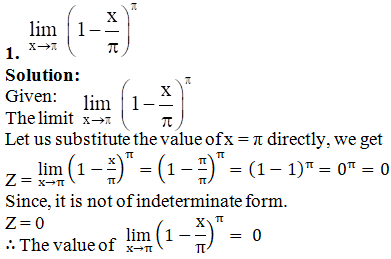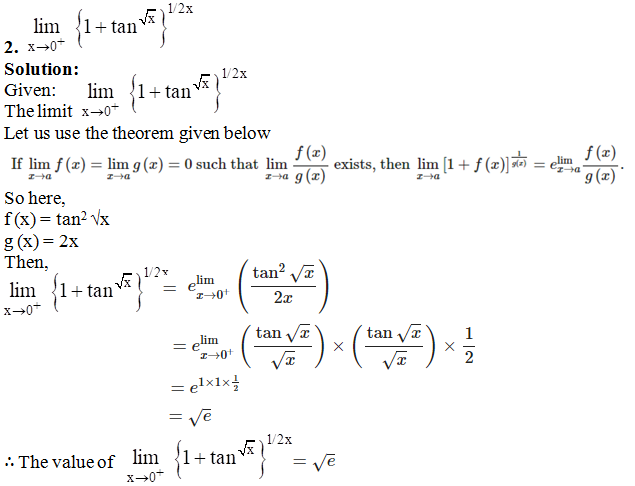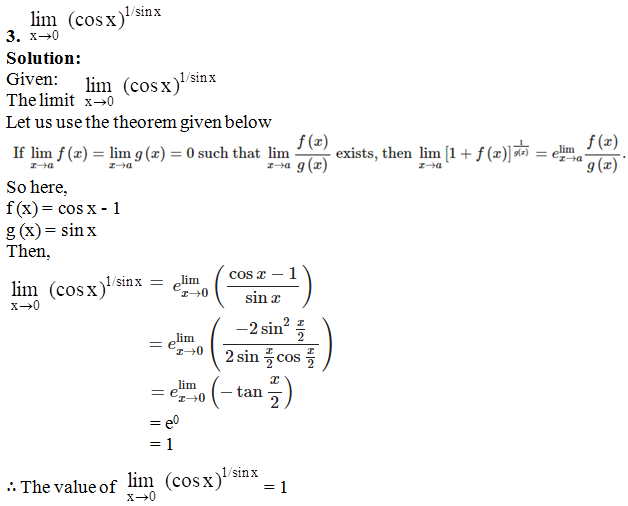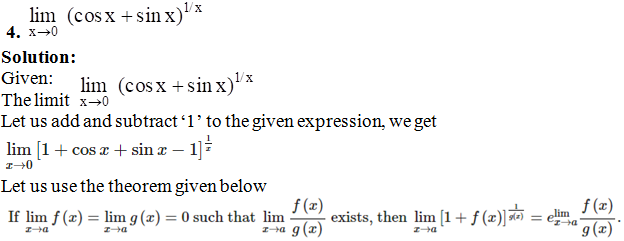So here,

f (x) = cos x + sin x – 1

g (x) = x

Then,